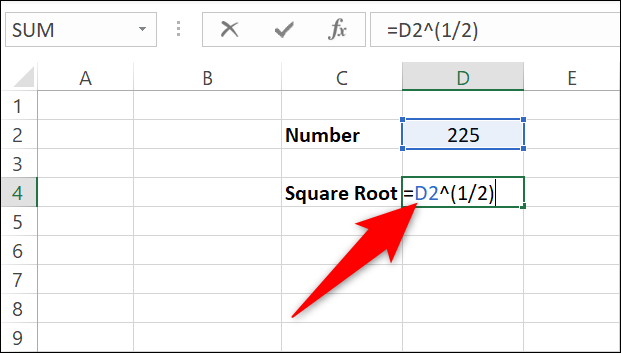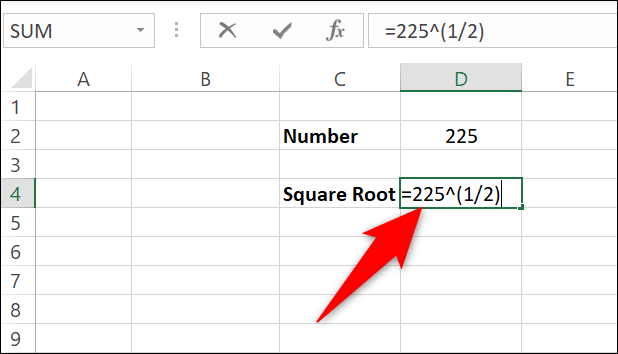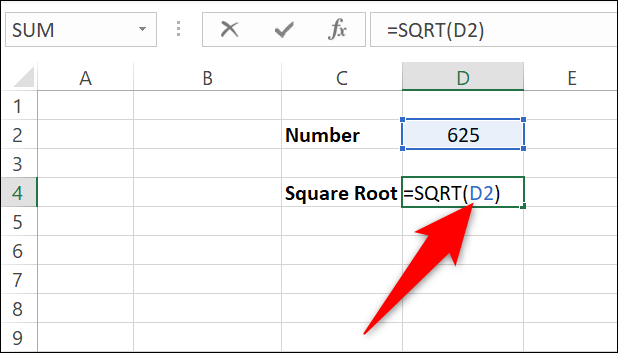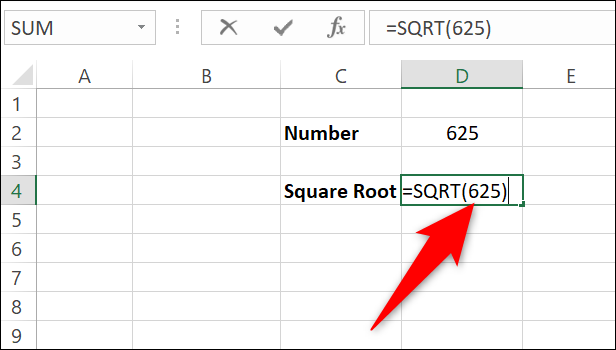# 如何在 Microsoft Excel 中计算平方根## 在 Excel 中使用插入符号计算平方根`=D2^(1/2)``=225^(1/2)`## 使用 SQRT 函数在 Excel 中计算平方根`=SQRT(D2)``=SQRT(625)`Excel 将计算平方根并将其显示在您选择的单元格中。## 额外提示：在 Excel 中插入平方根符号

`=UNICHAR(8730)&D2^(1/2)`

`=UNICHAR(8730)&SQRT(D2)`